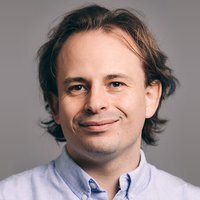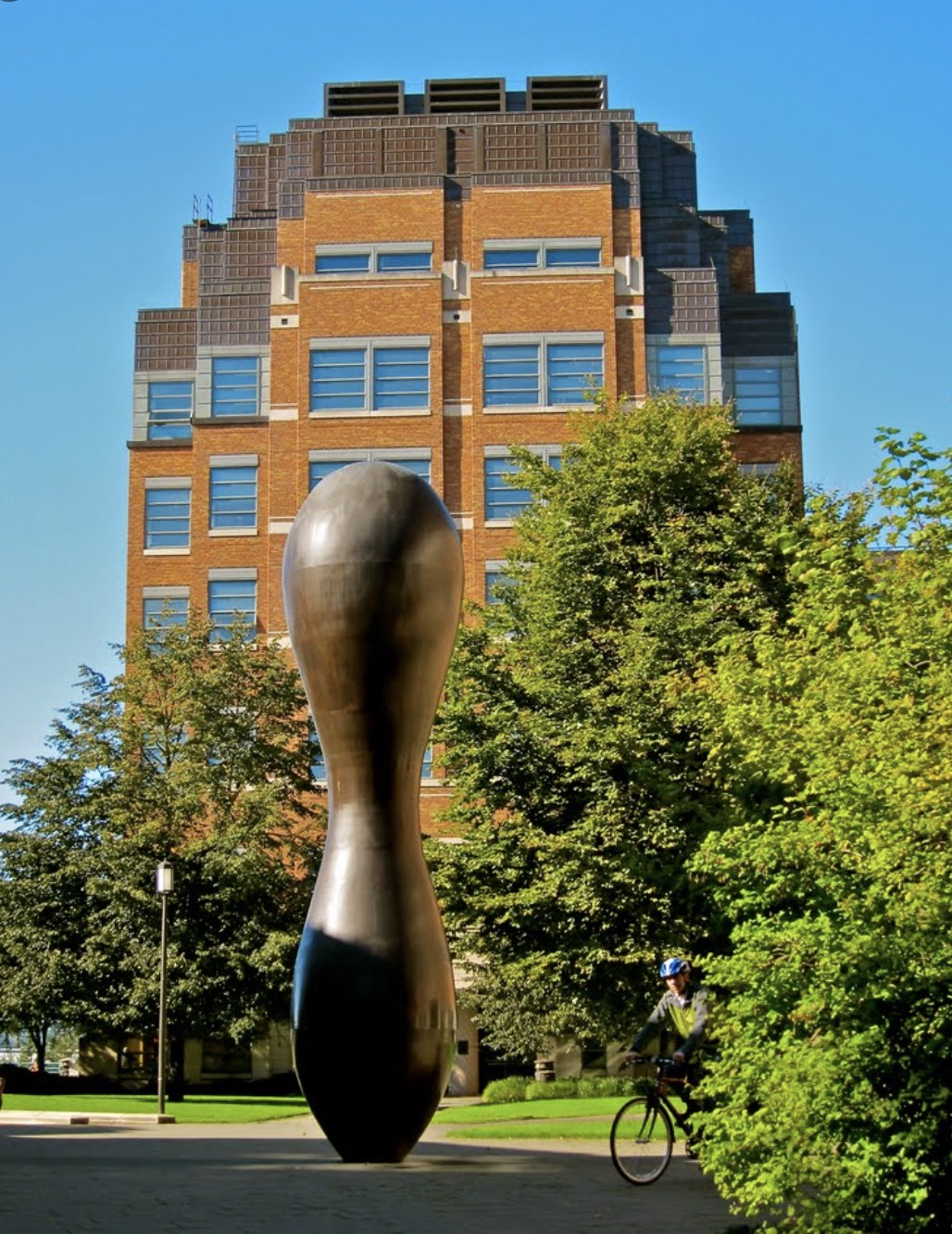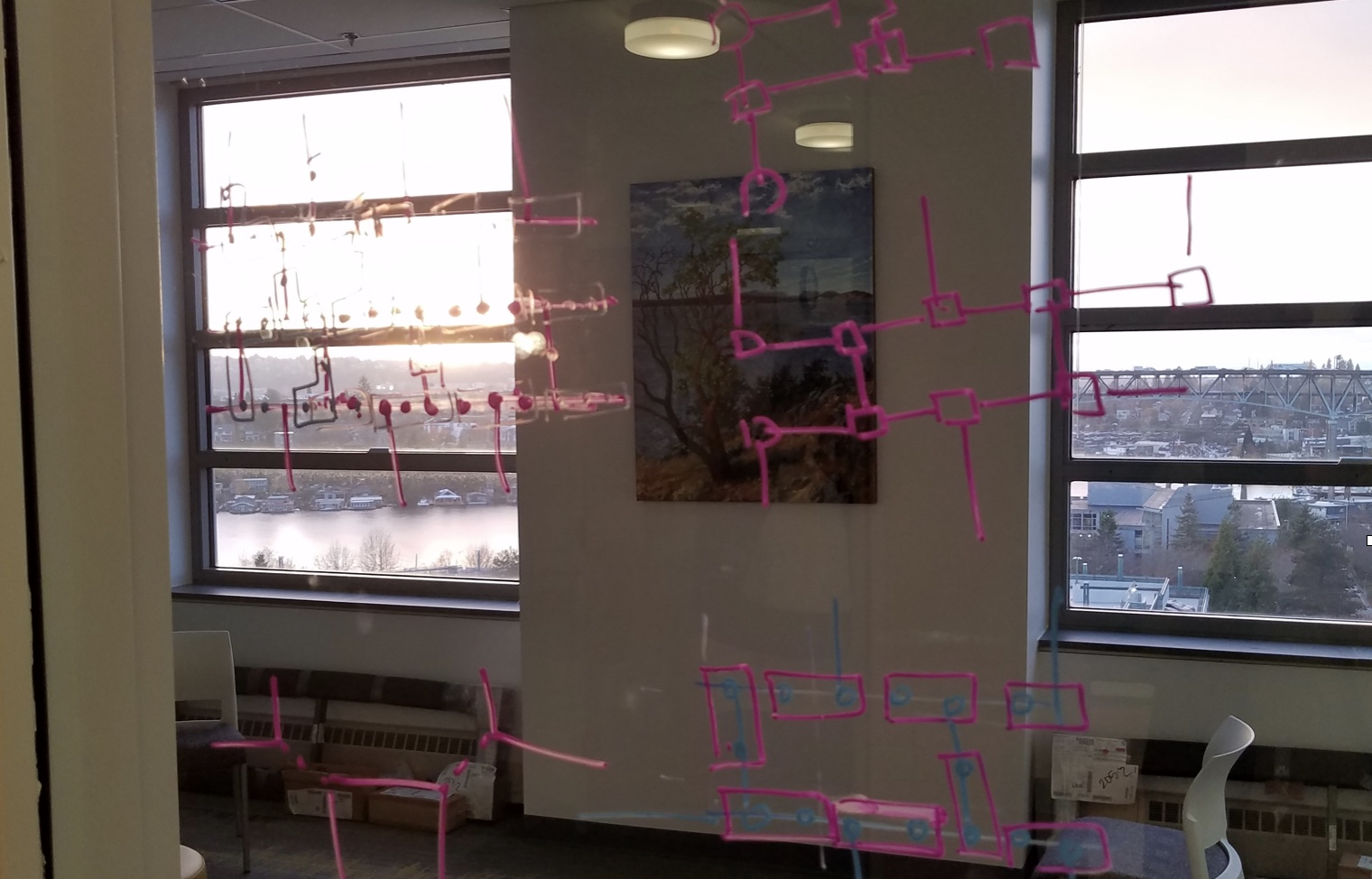# Events# Exponential quantum speedup in simulating coupled classical oscillators

Exponential quantum speedup in simulating coupled classical oscillators, by  Nathan Wiebe, University of TorontoExponential quantum speedup in simulating coupled classical oscillators

We present a quantum algorithm for simulating the classical dynamics of 2^n coupled oscillators (e.g., 2^n masses coupled by springs). Our approach leverages a mapping between the Schrödinger equation and Newton’s equation for harmonic potentials such that the amplitudes of the evolved quantum state encode the momenta and displacements of the classical oscillators. When individual masses and spring constants can be efficiently queried, and when the initial state can be efficiently prepared, the complexity of our quantum algorithm is polynomial in n, almost linear in the evolution time, and sublinear in the sparsity. As an example application, we apply our quantum algorithm to efficiently estimate the kinetic energy of an oscillator at any time. We show that any classical algorithm solving this same problem is inefficient and must make 2^(Ω(n)) queries to the oracle and, when the oracles are instantiated by efficient quantum circuits, the problem is BQP-complete. Thus, our approach solves a potentially practical application with an exponential speedup over classical computers. Finally, we show that under similar conditions our approach can efficiently simulate more general classical harmonic systems with 2^n modes.

Sold out!

Apr 26 2023
Expired!

### Time

1:30 pm - 2:30 pm### Location

UW, 15th and Pacific, Seattle
###### Website
https://phys.washington.edu/### Location 2### Location 3

University of Washington PHYS/ASTR Building, Seattle, WA 98195
Category